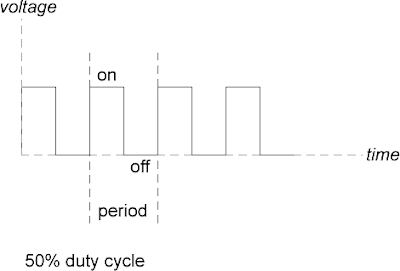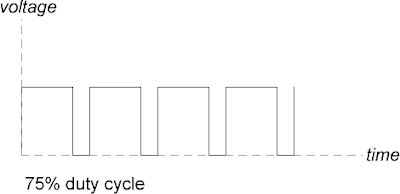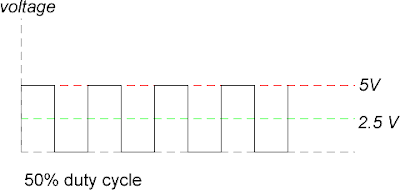## Friday, September 28, 2007

### PWM and You: A Quick Primer

Sebastian Tomczak, 28 September 2007

Duty Cycle
Consider a pulse wave – that is, a periodic waveform whose state can be either high or low (but no value in between) at any given point in time. Each pulse wave can be described in terms of frequency and amplitude, of course. But a third parameter exists for a pulse wave – that of duty cycle.The duty cycle of the waveform can be considered an amount, in percentage, of how much the waveform shape is high for a given period. So, if one considers the waveform below, it is possible to divide the period into two main sections – an ‘on’ state and an ‘off’ state. The duty cycle is given as the ratio between these two states, usually expressed as a percentage of the length of the ‘on’ state to the period as a whole. In the example below, it can be said that the duty cycle of the pulse wave is 50%, because the waveform is high for 50% of the period.Other duty cycles are of course possible as well, such as 75% and 25%.A DC voltage of 0V can be considered as having a duty cycle of 0%. A DC voltage of a constant voltage can be considered as having a duty cycle of 100%.

Pulse-Width Modulation
Pulse-width modulation is the act of changing the duty cycle of a periodic pulse waveform over time. This process does not involve changing the frequency or amplitude of the wave.

When a pulse-width modulated waveform is used to power certain devices (DC motors, LEDs etc), the width of the pulse (ie. the duty cycle) determines the voltage that is averaged out over time. This average voltage is the one that determines the power presented to the device. For DC motors, frequencies between 100Hz and 400Hz are suitable for PWM control.

The relationship between duty cycle and the average voltage “seen” by the receiving device is very direct. For example, if the duty cycle is 25%, then the average output voltage will be 25% of the voltage when the pulse wave is high.

In the diagrams below, a 5V power supply is assumed (for example, as found on the Arduino board). This power supply voltage is the same as what the microcontroller can output on its digital control pins, and is represented by the red line.

The green line represents the average voltage output, as “seen” by a DC motor (as found in a tape machine) for example.Arduino and PWM
When using pulse-width modulation with the Arduino board, the relevant control pin outputs a pulse waveform of a constant frequency of approximately 400Hz. The duty cycle of the wave is modulated from 0% to 100% in increments of 1/256.

Thus, the PWM output from the Arduino board can be used to control DC motors (such as those found inside tape machines, for example).

Consider the following Arduino code and hardwire connections. The outcome of the code and the hardwiring is that the current position of the pot determines the present duty cycle of the waveform created by the digital control pin used for pulse-width modulation. Thus, it is possible for the Arduino to control the speed of a DC motor quite easily by turning a pot.

Hardwiring:
• ULN2003 PIN 8 to GND
• ULN2003 PIN 9 to 5V
• ULN2003 PIN 1 to Arduino digital control pin 9
• ULN2003 PIN 16 to one terminal of the motor
• the other terminal of the motor to 5V
• one of the outer legs of the potentiometer to 5V
• the other outer leg of the potentiometer to GND
• the middle leg of the potentiometer to Arduino analog in 0

Arduino Code:

`byte data;void setup() {}void loop () {data = analogRead(0) / 4;analogWrite(9, data);delay(5);}`